Courses

# Short Notes - Work and Energy Class 9 Notes | EduRev

## Class 9 : Short Notes - Work and Energy Class 9 Notes | EduRev

The document Short Notes - Work and Energy Class 9 Notes | EduRev is a part of the Class 9 Course Class 9 Science by VP Classes.
All you need of Class 9 at this link: Class 9

Facts that Matter

• Work: When a force acts on an object and the object shows displacement, the force has done work on the object.
Two conditions need to be satisfied for work to be done:
(i) A force should act on the object
(ii) The object must be displaced
Work = Force × Displacement = F × S
Unit of work done = Joule = Newton × metre

• 1 Joule work is said to be done when 1 Newton force is applied on an object and it shows the displacement by 1 meter. (Case I)
If displacement is in the direction of the force
W = F × s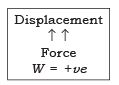If displacement is in the direction opposite to the force
W = − F × s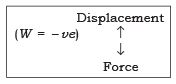(Case II) If displacement is perpendicular to the force, work done is zero.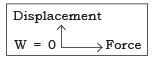• Energy The capacity of a body to do work is called the energy of the body.

Unit of energy = Joules
1 kJ = 1000 J

•  Forms of Energy: The various forms of energy are potential energy, kinetic energy, heat energy, chemical energy, electrical energy and light energy.
• Kinetic Energy: Energy possessed by a body due to its motion. Kinetic energy of an object increases with its speed.

Kinetic energy of body moving with a certain velocity = work done on it to make it acquire that velocity.

• Derivation
Let an object of mass m, moves with uniform velocity u, let us displace it by s, due to constant force F, acting on it
∴ Work done → W = F × s ... (i)
due to force the velocity changes to v, and the acceleration produced is a
∴  Relationship between v, u, a and s : v2 − u2 = 2as

∴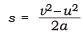... (ii) and
F = ma             ... (iii)
Substitute (ii) and (iii) in (i), we get W = F × s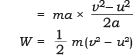if u = 0, (object starts at rest)
∴         W = (1/2) mv
Work done = Change in kinetic energy
∴       Ek = (1/2)mv2

• Potential Energy The energy possessed by a body due to its position or shape is called its potential energy.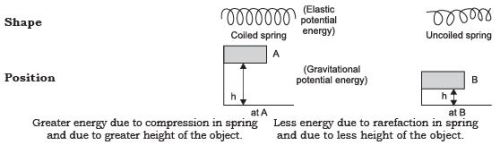• Gravitational Potential Energy: (GPE) When an object is raised through a height, work is said to be done on it against gravity.

The energy possessed by such an object is called the gravitational potential energy.
∴ GPE = work done in raising a body from the ground to a point against gravity.

• Derivation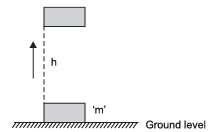Consider a body with mass m, raised through a height h, from the ground, Force required to raise the object = weight of object mg.

Object gains energy equal to the work done on it.
∴ Work done on the object against gravity is W.
∴  W = force × displacement = mg × h
W = mgh
∴              PE = mgh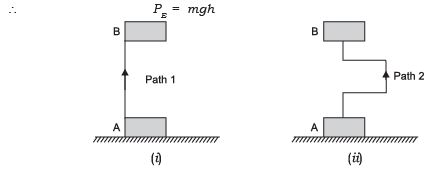Work done in both the cases (i) and (ii) is same as a body is raised from position A to B, even if the path taken is different but the height attained is the same.

• Mechanical Energy: The sum of kinetic energy and potential energy is called mechanical energy.
• Law of Conservation of Energy: Energy can neither be created nor destroyed, it can only be transformed from one form to another. The total energy before and after transformation remains the same.

Case (i)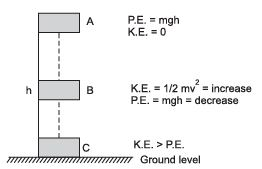Potential energy + Kinetic energy = Constant (Mechanical energy)

A body of mass ‘m’ is raised to height ‘h’ at A its potential energy is maximum and kinetic energy is 0 as it is stationary.
When body falls at B, h is decreasing hence potential energy decreases and ‘v’ is increasing hence kinetic energy is increasing.
When the body is about to reach the ground level, h = 0, v will be maximum hence kinetic energy > potential energy

∴ Decrease in potential energy = Increase in kinetic energy

This shows the continual transformation of gravitational potential energy into kinetic energy.

• Power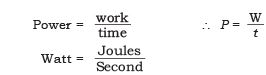1 kilowatt = 1000 watts
1 kilowatt = 1000 J/s

• Commercial Unit of Energy
Commercial unit of energy = 1 kilowatt hour (kWh)
∴ 1 kWh = 1 kilowatt × 1 hour = 1000 watt × 3600 seconds
= 3600000 Joule (watt × second)
1 kWh = 3.6 × 106 J.
∴                  1 kWh = 1 unit

The energy used in one hour at the rate of 1kW is called 1kWh.

Offer running on EduRev: Apply code STAYHOME200 to get INR 200 off on our premium plan EduRev Infinity!

75 docs|14 tests

,

,

,

,

,

,

,

,

,

,

,

,

,

,

,

,

,

,

,

,

,

;